International
Tables for
Crystallography
Volume B
Reciprocal space
Edited by U. Shmueli

International Tables for Crystallography (2010). Vol. B, ch. 2.5, pp. 304-306   | 1 | 2 |

## Section 2.5.2.7. Imaging of very thin and weakly scattering objects

J. M. Cowleya

#### 2.5.2.7. Imaging of very thin and weakly scattering objects

| top | pdf |

• (a) The weak-phase-object approximation. For sufficiently thin objects, the effect of the object on the incident-beam amplitude may be represented by the transmission function (2.5.2.16)given by the phase-object approximation. If the fluctuations,, about the mean value of the projected potential are sufficiently small so that, it is possible to use the weak-phase-object approximation (WPOA)whereis referred to the average value,. The assumption that only first-order terms inneed be considered is the equivalent of a single-scattering, or kinematical, approximation applied to the two-dimensional function, the projected potential of (2.5.2.16). From (2.5.2.42), the image intensity (2.5.2.35)becomeswhere the spread function s(xy) is the Fourier transform of the imaginary part of T(uv), namely.

The optimum imaging condition is then found, following Scherzer (1949), by specifying that the defocus should be such thatis close to unity for as large a range ofas possible. This is so for a negative defocus such thatdecreases to a minimum of aboutbefore increasing to zero and higher as a result of the fourth-order term of (2.5.2.33)(see Fig. 2.5.2.3). This optimum, Scherzer defocus' value is given byor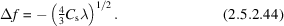Figure 2.5.2.3 | top | pdf |The functions, the phase factor for the transfer function of a lens given by equation (2.5.2.33), andfor the Scherzer optimum defocus condition, relevant for weak phase objects, for which the minimum value ofis.

The resolution limit is then taken as corresponding to the value of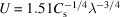whenbecomes zero, before it begins to oscillate rapidly with U. The resolution limit is then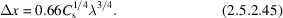For example, for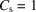mm andÅ (200 keV),Å.

Within the limits of the WPOA, the image intensity can be written simply for a number of other imaging modes in terms of the Fourier transformsandof the real and imaginary parts of the objective-lens transfer function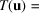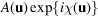, where r and u are two-dimensional vectors in real and reciprocal space, respectively.

For dark-field TEM images, obtained by introducing a central stop to block out the central beam in the diffraction pattern in the back-focal plane of the objective lens,Here, as in (2.5.2.42),should be taken to imply the difference from the mean potential value,.

For bright-field STEM imaging with a very small detector placed axially in the central beam of the diffraction pattern (2.5.2.39)on the detector plane, the intensity, from (2.5.2.41), is given by (2.5.2.43).

For a finite axially symmetric detector, described by, the image intensity iswhereis the Fourier transform of(Cowley & Au, 1978).

For STEM with an annular dark-field detector which collects all electrons scattered outside the central spot of the diffraction pattern in the detector plane, it can be shown that, to a good approximation (valid except near the resolution limit)Sinceis the intensity distribution of the electron probe incident on the specimen, (2.5.2.48)is equivalent to the incoherent imaging of the function.

Within the range of validity of the WPOA or, in general, whenever the zero beam of the diffraction pattern is very much stronger than any diffracted beam, the general expression (2.5.2.36)for the modifications of image intensities due to limited coherence may be conveniently approximated. The effect of integrating over the variables, may be represented by multiplying the transfer function T(u, v) by so-called envelope functions' which involve the Fourier transforms of the functionsand.

For example, ifis approximated by a Gaussian of width(atof the maximum) centred atandis a circular aperture function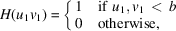the transfer functionfor coherent radiation is multiplied bywhere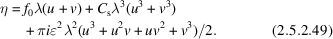• (b) The projected charge-density approximation. For very thin specimens composed of moderately heavy atoms, the WPOA is inadequate. Within the region of validity of the phase-object approximation (POA), more complicated relations analagous to (2.5.2.43)to (2.5.2.47)may be written. A simpler expression may be obtained by use of the two-dimensional form of Poisson's equation, relating the projected potential distributionto the projected charge-density distribution. This is the PCDA (projected charge-density approximation) (Cowley & Moodie, 1960),This is valid for sufficiently small values of the defocus, provided that the effects of the spherical aberration may be neglected, i.e. for image resolutions not too close to the Scherzer resolution limit (Lynch et al., 1975). The functionincludes contributions from both the positive atomic nuclei and the negative electron clouds. For underfocus (negative), single atoms give dark spots in the image. The contrast reverses with defocus.

### References

Cowley, J. M. & Au, A. Y. (1978). Image signals and detector configurations for STEM. In Scanning Electron Microscopy, Vol. 1, pp. 53–60. AFM O'Hare: SEM Inc.
Cowley, J. M. & Moodie, A. F. (1960). Fourier images. IV. The phase grating. Proc. Phys. Soc. London, 76, 378–384.
Lynch, D. F., Moodie, A. F. & O'Keefe, M. A. (1975). n-Beam lattice images. V. The use of the charge-density approximation in the interpretation of lattice images. Acta Cryst. A31, 300–307.
Scherzer, O. (1949). The theoretical resolution limit of the electron microscope. J. Appl. Phys. 20, 20–29.